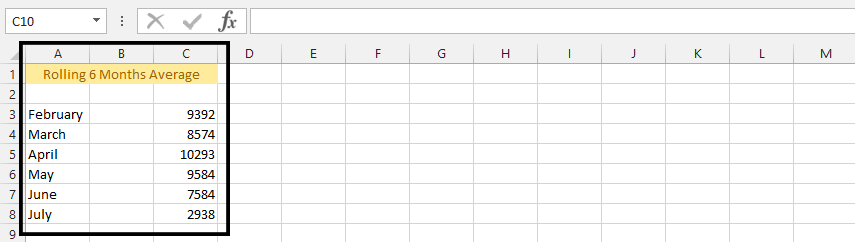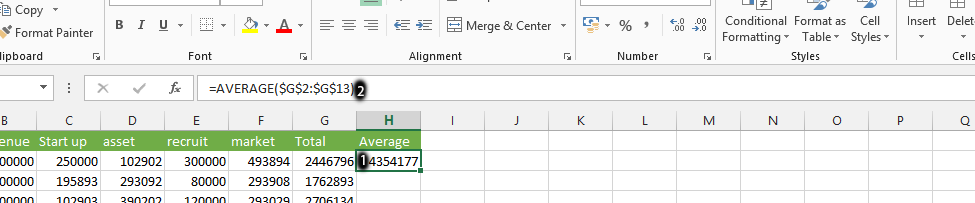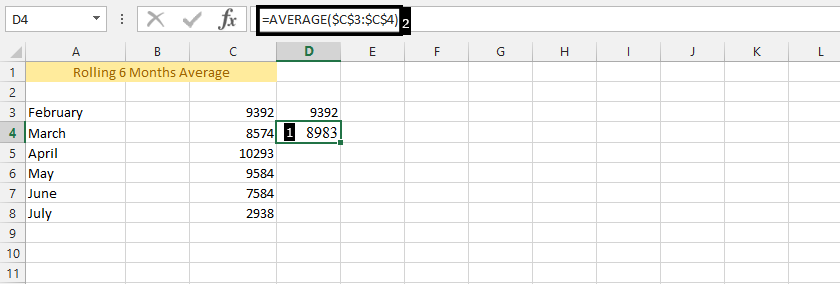#### How to Calculate Rolling Average in Excel

A rolling average is a type of moving average that calculates the average of a set of data points over a specified period. The period can be fixed or variable, and it is typically used to smooth out fluctuations in the data.

## How to calculate a rolling average in Excel

There are two ways to calculate a rolling average in Excel: using formulas and using the Data Analysis ToolPak.

## Using formulas

### Using Average function

To calculate a rolling 6 months average in Excel, you can follow these steps:Click on an empty cell, and type =AVERAGE(\$C\$3:\$C\$3).Click on cell D4, then write =AVERAGE(\$C\$3:\$C\$4).To copy the formula to the remaining cells, select the cell that contains the formula and drag the fill handle down to the last cell in the range.

The formula will be copied to the remaining cells and the rolling average will be calculated for each month.

### Using INDEX function

Alternatively, you can use the INDEX function to select the range of cells:

1. Select the cell where you want to display the rolling average.
2. Enter the formula: =AVERAGE(INDEX(C:C,ROW()-n+1):INDEX(C:C,ROW()))Where:
• C:C is the column that contains your data.
• n is the number of data points you want to include in the rolling average.

For example, if you want to calculate a 6-month rolling average, the formula would be: =AVERAGE(INDEX(C:C,ROW()-5+1):INDEX(C:C,ROW()))

3. Press Enter to calculate the rolling average for the first set of data.
4. Copy the formula to the remaining cells in the column.

Note that the formulas will adjust automatically as you copy them to the rest of the cells in the column, and the rolling average will update accordingly.

## Using the Data Analysis ToolPak

Excel also has a built-in tool called the Data Analysis tool that can be used to calculate rolling and moving averages. To use the Data Analysis tool, follow these steps:

Click on the Data tab. In the Analysis group, click on the Data Analysis button.

In the Data Analysis dialog box, select Moving Average from the list of tools.

In the Moving Average dialog box, specify the following options:

• The range of cells that contain the data you want to average.
• The number of data points you want to include in the average.
• The output range where you want the results to be displayed.

The Data Analysis tool will calculate the rolling or moving average and display the results in the output range you specified.